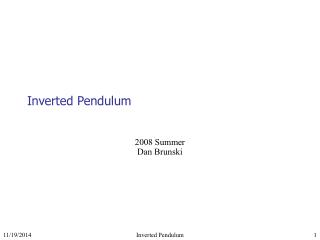DownloadDownload PresentationInverted Pendulum

# Inverted Pendulum

Télécharger la présentation## Inverted Pendulum

- - - - - - - - - - - - - - - - - - - - - - - - - - - E N D - - - - - - - - - - - - - - - - - - - - - - - - - - -
##### Presentation Transcript

1. Inverted Pendulum 2008 Summer Dan Brunski Inverted Pendulum

2. Theory Mathieu equation • A pendulum with an oscillating base can be described by the Mathieu equation • Regions of stability exist in the Mathieu equation for δ < 0 • These regions correspond to an inverted pendulum position Regions of stability of the Mathieu equation Regular pendulum Inverted pendulum Inverted Pendulum

3. Equation Development b(t) • The coordinates of a pendulum with mass m on an oscillating base b(t) are given by • The Lagrangian is then • Using the Euler-Lagrange equation and substituting in b(t), the equation of motion is • Making the following substitutions, the Mathieu equation is obtained Inverted Pendulum

4. What Didn’t Work • Pendulums powered by either Black and Decker jigsaw or Dewalt reciprocating saw • Pendulum dimensions: • 16-1/4” x 1-1/4” x 1/8” • Length measured from pivot center to end • Lengths down to 6-3/4” • Tried adding weights to various places • Either the frequency wasn’t high enough, amplitude not great enough, modes of vibration in unintended directions, or a function of all three Inverted Pendulum

5. What Worked • Short piece of straw (44.5mm from pivot to end) on a Pasco SF-9324 wave driver • Foam pad under wave driver to keep noise down and stop it from bouncing around • Function generator signal amplified to wave driver limit of 16Vpp • Pivot made from 0.021” steel wire welded to a washer • Confirmed stable settings: • 41Hz – 77Hz • 16Vpp ± 0.5V • 5.39Vrms ± 0.1V • 377mA ± 1mA • 4mm ± 0.5mm amplitude Inverted Pendulum

6. Wiring Schematic Function Generator Amplifier Frequency Counter Ammeter Voltmeter Wave Driver Oscilloscope • Ammeter, Voltmeter, and oscilloscope are not necessary, but recommended to prevent damaging the wave driver Inverted Pendulum